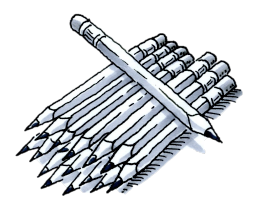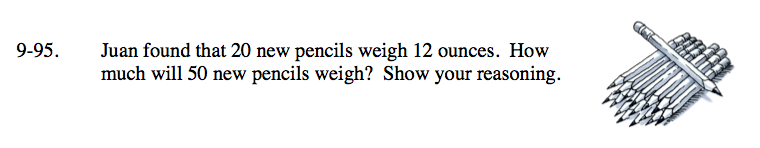Home > ACC7 > Chapter cc39 > Lesson cc39.2.3 > Problem9-95

9-95.Juan found that 20 new pencils weigh 12 ounces. How much will 50 new pencils weigh? Show your reasoning. Homework Help ✎Notice that this is a proportion problem. The weight per pencil does not change as the number of pencils increases or decreases.

Set up equivalent ratios that compare the number of pencils to the weight of the pencils.

$\frac{20\;\text{pencils}}{12\;\text{ounces}} = \frac{50\; \text{pencils}}{\it x}$

Solve for x.

x = 30 ounces